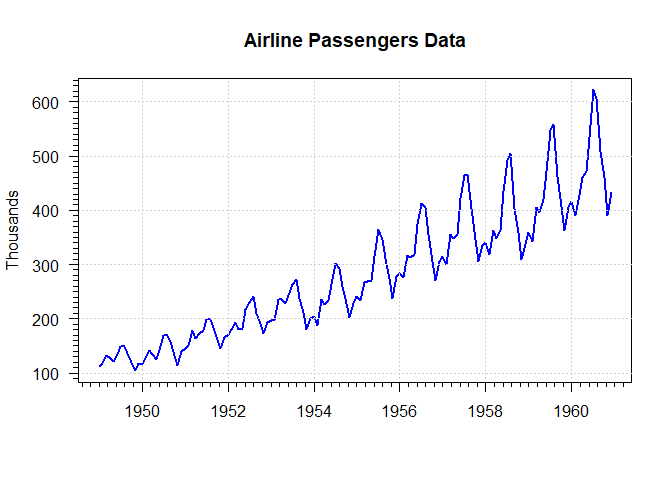Purpose: Enable students to compute typical time-series transformations, estimate and interpret AR(1) models.

# Introduction

For the first part of this exercise we are considering out usual Airline Passengers data set. The exercises follow closely the R codes from this week’s lecture.

Here’s a plot of this data:# Question 1

1 Point

Recall how in the lecture we computed the percentage change at the compound annual rate of real GDP growth (DIFA.P) as follows:

$\mbox{DIFA.P} = \left(\left(\frac{x_t}{x_{t-1}}\right)^{npy}-1\right)\times 100$

where $$npy$$ is the frequency per year. In most of the applications that we considered $$npy$$ = 4 for quarterly data and $$npy$$ = 12 for monthly data.

Compute the DIFA.P for the Airline Passenger data. Plot DIFA.P in a diagram with a line width of 2. Add a horizontal line at zero in black with a line width of 1. Add a horizontal red dashed line a the mean of DIFA.P.

What is the mean of DIFA.P?

# Question 2

1 Point

Recall how in the lecture we computed the period to period percentage change of real GDP growth (DIFF.P) as follows:

$\mbox{DIFF.P} = \left(\left(\frac{x_t}{x_{t-1}}\right)-1\right)\times 100$

where $$npy$$ is the frequency per year. In most of the applications that we considered $$npy$$ = 4 for quarterly data (such as in the lecture with U.S. real GDP) and $$npy$$ = 12 for monthly data.

Compute the DIFF.P for the Airline Passenger data. Plot DIFF.P in a diagram with a line width of 2. Add a horizontal line at zero in black with a line width of 1. Add a horizontal red dashed line a the mean of DIFF.P.

What is the mean of DIFF.P?

# Question 3

1 Point

Recall how in the lecture we computed the year over year percentage change (YRYR.P) as follows:

$\mbox{YRYR.P} = \left(\left(\frac{x_t}{x_{t-npy}}\right)-1\right)\times 100$

where $$npy$$ is the frequency per year. In most of the applications that we considered $$npy$$ = 4 for quarterly data and $$npy$$ = 12 for monthly data.

Compute the YRYR.P for the Airline Passenger data. Plot YRYR.P in a diagram with a line width of 2. Add a horizontal line at zero in black with a line width of 1. Add a horizontal red dashed line a the mean of YRYR.P.

What is the mean of YRYR.P?

# Question 4

1 Point

In the lecture we computed the period to period difference change (DIFF.D) as follows:

$\mbox{DIFF.D} = x_t - x_{t-1}$

where $$npy$$ is the frequency per year. In most of the applications that we considered $$npy$$ = 4 for quarterly data and $$npy$$ = 12 for monthly data.

Compute the DIFF.D for the Airline Passenger data. Plot DIFF.D in a diagram with a line width of 2. Add a horizontal line at zero in black with a line width of 1. Add a horizontal red dashed line a the mean of DIFF.D.

What is the mean of DIFF.D?

# Question 5

1 Point

In the lecture we computed the year over year difference change (YRYR.D) as follows:

$\mbox{YRYR.D} = x_t - x_{t-npy}$

where $$npy$$ is the frequency per year. In most of the applications that we considered $$npy$$ = 4 for quarterly data and $$npy$$ = 12 for monthly data.

Compute the YRYR.D for the Airline Passenger data. Plot YRYR in a diagram with a line width of 2. Add a horizontal line at zero in black with a line width of 1. Add a horizontal red dashed line a the mean of YRYR.D.

What is the mean of YRYR.D?

# Question 6

1 Point

Recall how in the lecture we computed the period over period log change at an annual rate (DIFA.L) as follows:

$\mbox{DIFA.L} = \mbox{ln} \left(\frac{x_t}{x_{t-1}}\right)\times npy \times 100$

where $$npy$$ is the frequency per year. In most of the applications that we considered $$npy$$ = 4 for quarterly data and $$npy$$ = 12 for monthly data.

Compute the DIFA.L for the Airline Passenger data. Plot DIFA.L in a diagram with a line width of 2. Add a horizontal line at zero in black with a line width of 1. Add a horizontal red dashed line a the mean of DIFA.L.

What is the mean of DIFA.L?

# Question 7

1 Point

Recall how in the lecture we computed the period to period log change (DIFF.L) as follows:

$\mbox{DIFF.L} = \mbox{ln} \left(\frac{x_t}{x_{t-1}}\right) \times 100$

where $$npy$$ is the frequency per year. In most of the applications that we considered $$npy$$ = 4 for quarterly data and $$npy$$ = 12 for monthly data.

Compute the DIFF.L for the Airline Passenger data. Plot DIFF.L in a diagram with a line width of 2. Add a horizontal line at zero in black with a line width of 1. Add a horizontal red dashed line a the mean of DIFF.L.

What is the mean of DIFF.L?

# Question 8

1 Point

Finally, we computed the year to year log change (YRYR.L) as follows:

$\mbox{YRYR.L} = \mbox{ln} \left(\frac{x_t}{x_{t-npy}}\right) \times 100$

where $$npy$$ is the frequency per year. In most of the applications that we considered $$npy$$ = 4 for quarterly data and $$npy$$ = 12 for monthly data.

Compute the YRYR.L for the Airline Passenger data. Plot YRYR.L in a diagram with a line width of 2. Add a horizontal line at zero in black with a line width of 1. Add a horizontal red dashed line a the mean of YRYR.L.

What is the mean of YRYR.L?

# Question 9

1 Point

Using the series DIFF.L constructed above, estimate an autoregressive model of the type:

$y_t = \alpha + \rho \cdot y_{t-1} + \varepsilon_t$

The following lines in R might be helpful:

install.packages("dynlm")
library(dynlm)

mod.ar1 <- dynlm(DIFF.L~L(DIFF.L,1))
summary(mod.ar1)
coef(summary(mod.ar1))
coef(summary(mod.ar1))
coef(summary(mod.ar1))

What is the autoregressive coefficient $$\rho$$?

# Question 10

1 Point

What is the unconditonal mean of the AR(1) progress that you estimated in Question 9.

# Optional

How does the answer to Question 10 compare to the answer to Question 7?

Think about how you would determine whether an AR(1) model is appropriate for the transformed Airline Passenger data set.